NEET  >  Test: Gravitation - 1

# Test: Gravitation - 1

Test Description

## 20 Questions MCQ Test Physics Class 11 | Test: Gravitation - 1

Test: Gravitation - 1 for NEET 2022 is part of Physics Class 11 preparation. The Test: Gravitation - 1 questions and answers have been prepared according to the NEET exam syllabus.The Test: Gravitation - 1 MCQs are made for NEET 2022 Exam. Find important definitions, questions, notes, meanings, examples, exercises, MCQs and online tests for Test: Gravitation - 1 below.
Solutions of Test: Gravitation - 1 questions in English are available as part of our Physics Class 11 for NEET & Test: Gravitation - 1 solutions in Hindi for Physics Class 11 course. Download more important topics, notes, lectures and mock test series for NEET Exam by signing up for free. Attempt Test: Gravitation - 1 | 20 questions in 28 minutes | Mock test for NEET preparation | Free important questions MCQ to study Physics Class 11 for NEET Exam | Download free PDF with solutions
 1 Crore+ students have signed up on EduRev. Have you?
Test: Gravitation - 1 - Question 1

### According to Kepler’s Law of orbits:

Detailed Solution for Test: Gravitation - 1 - Question 1
• The orbit of a planet around the Sun (or of a satellite around a planet) is not a perfect circle. It is an ellipse—a “flattened” circle.
• The Sun (or the centre of the planet) occupies one focus of the ellipse. A focus is one of the two internal points that help determine the shape of an ellipse.
• The distance from one focus to any point on the ellipse and then back to the second focus is always the same.

Kepler's Law of Orbits:Test: Gravitation - 1 - Question 2

### Escape speed from the earth is the

Detailed Solution for Test: Gravitation - 1 - Question 2

The escape velocity is the minimum velocity required to leave a planet or moon. For a rocket or other object to leave a planet, it must overcome the pull of gravity.

Vescape = √2GM/R
Vescape = 11184 m/sec approximate to 11.2 km/sec

Test: Gravitation - 1 - Question 3

### According to Kepler’s Law of areas,

Detailed Solution for Test: Gravitation - 1 - Question 3
• Kepler law of areas describes the speed of a planet travelling in an elliptical orbit around the sun. A planet’s orbital speed changes, depending on how far it is from the Sun.
• The closer a planet is to the Sun, the stronger the Sun’s gravitational pull on it, and the faster the planet moves.
• The farther it is from the Sun, the weaker the Sun’s gravitational pull, and the slower it moves in its orbit.
• So, statement of Kepler's law of area is "The line joining the sun to the planet sweeps out equal areas in equal interval of time". i.e. areal velocity is constant.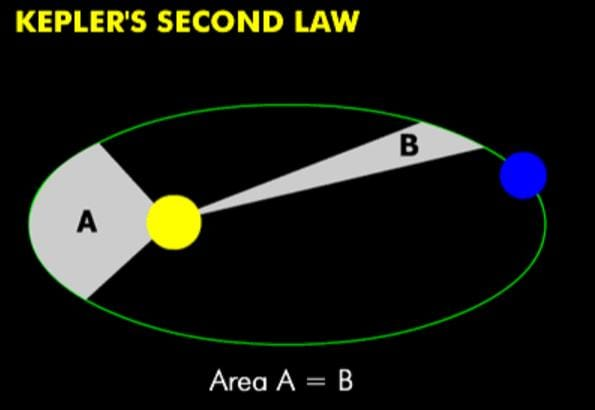Test: Gravitation - 1 - Question 4

The total energy of a circularly orbiting satellite is

Detailed Solution for Test: Gravitation - 1 - Question 4
• When a satellite revolves around a planet in its orbit, it possesses both potential energy (due to its position against the gravitational pull of earth) and kinetic energy (due to orbital motion).
• The total energy of a circularly orbiting satellite is thus negative, with the potential energy being negative but twice is the magnitude of the positive kinetic energy.
Test: Gravitation - 1 - Question 5

According to Kepler’s Law of periods, The  _______________ of the time period of revolution of a planet is proportional to the cube of the  ___________ of the ellipse traced out by the planet

Detailed Solution for Test: Gravitation - 1 - Question 5

Kepler's 3rd law is a mathematical formula. It means that if you know the period of a planet's orbit (T = how long it takes the planet to go around the Sun), then you can determine that planet's distance from the Sun (a is the length of the semimajor axis of the planet's orbit)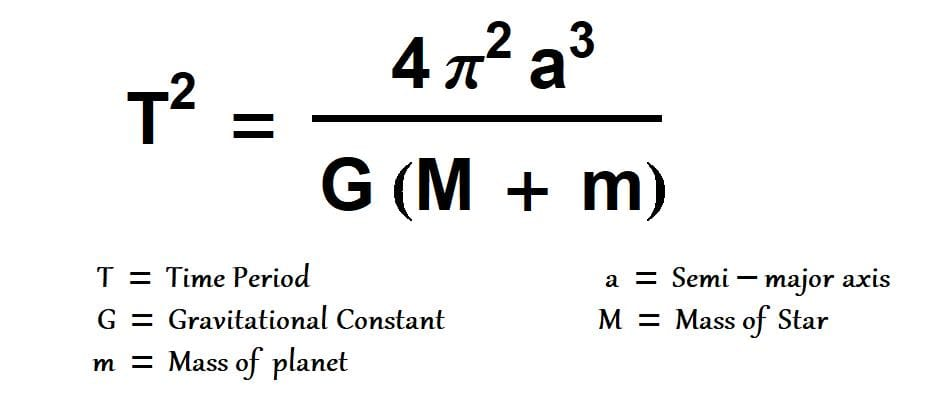Test: Gravitation - 1 - Question 6

A ‘central’ force is always directed

Detailed Solution for Test: Gravitation - 1 - Question 6
• The motion of a particle under a central force F always remains in the plane defined by its initial position and velocity. This may be seen by symmetry.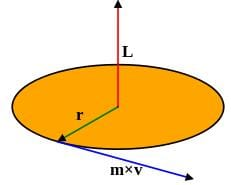• Since the position r, velocity v and force F all lie in the same plane, there is never an acceleration perpendicular to that plane, because that would break the symmetry between "above" the plane and "below" the plane.
• To demonstrate this mathematically, it suffices to show that the angular momentum of the particle is constant. This angular momentum L is defined by the equation r×r×mv
Test: Gravitation - 1 - Question 7

The acceleration due to gravity at the North Pole of Neptune is approximately 10.7 m/s2. Neptune has mass 1.0 x 1026 kg and radius 2.5 x 104 km and rotates once around its axis in about 16 h. What is the gravitational force on a 5.0-kg object at the north pole of Neptune?

Detailed Solution for Test: Gravitation - 1 - Question 7

Gravitational Force:
F = mg
⇒ F = 5×10.7 = 53.5 N

Test: Gravitation - 1 - Question 8

To find the resultant gravitational force acting on the particle m due to a number of masses we need to use:

Detailed Solution for Test: Gravitation - 1 - Question 8

According to properties of gravitational force, gravitational force between the particles is independent of the presence or absence of other particles; so the principle of superposition is valid i.e. force on a particle due to number of particles is the resultant of forces due to individual particles.

Test: Gravitation - 1 - Question 9

For Geostationary Satellites

Detailed Solution for Test: Gravitation - 1 - Question 9

The satellite which appear stationary relative to earth, such satellites are called geostationary satellites and will have a period of rotation as the period of rotation of earth i.e  24 Hrs.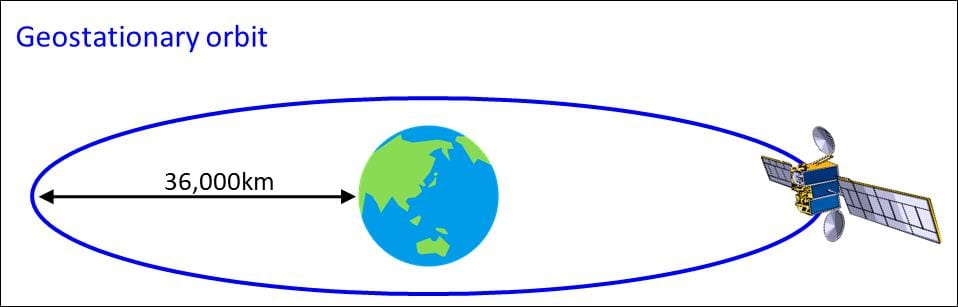Test: Gravitation - 1 - Question 10

The acceleration due to gravity at the North Pole of Neptune is approximately 10.7 x  m/s2. Neptune has mass 1.0 x 1026 kg and radius 2.5 x 104 km and rotates once around its axis in about 16 h. What is the apparent weight a 5.0-kg object at Neptune’s equator?

Detailed Solution for Test: Gravitation - 1 - Question 10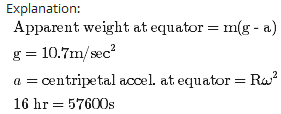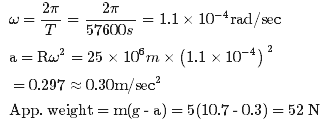Test: Gravitation - 1 - Question 11

The force of attraction between a hollow spherical shell of uniform density and a point mass situated outside is just as if the entire mass of the shell is

Detailed Solution for Test: Gravitation - 1 - Question 11
• According to Shell's theorem, If a particle of mass m is located outside a spherical shell of mass M at, for instance, point P, the shell attracts the particle as though the mass of the shell were concentrated at its centre.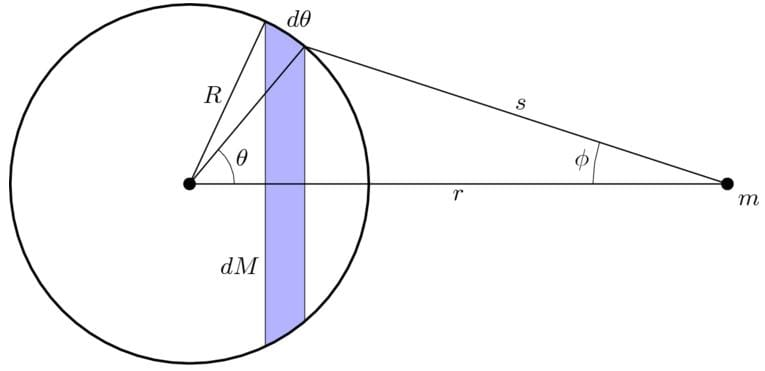• Thus, as far as the gravitational force acting on a particle outside the shell is concerned, a spherical shell acts no differently from the solid spherical distributions of mass.
Test: Gravitation - 1 - Question 12

Geostationary Satellites

Detailed Solution for Test: Gravitation - 1 - Question 12
• The orbital velocity of the satellite depends on its altitude above Earth.
• The nearer Earth, the faster the required orbital velocity. At an altitude of 200 kilometers, the required orbital velocity is just over 27,400 kph.
• To maintain an orbit that is 35,786 km above Earth, the satellite must orbit at a speed of about 11,300 kph. That orbital speed and distance permits the satellite to make one revolution in 24 hours.
• To achieve his speed, Judging from the mass of fuel needed, it stands to reason that you'd use a more powerful launch vehicle with a bi-propellant upper stage to get the satellite to GEO.
Test: Gravitation - 1 - Question 13

The force of attraction due to a hollow spherical shell of uniform density, on a point mass situated inside it, is

Detailed Solution for Test: Gravitation - 1 - Question 13
• The gravitational force acting by a spherically symmetric shell upon a point mass inside it, is the vector sum of gravitational forces acted by each part of the shell, and this vector sum is equal to zero.
• That is, a mass m within a spherically symmetric shell of mass M will feel no net force.
Test: Gravitation - 1 - Question 14

Suppose there existed a planet that went around the sun twice as fast as the earth. What would be its orbital size as compared to that of the earth?

Detailed Solution for Test: Gravitation - 1 - Question 14

Time period of revolution of earth around sun Te= 1 Year

Time period of revolution of planet around sun,Tp=0.5 Year

Orbital size of earth, re= 1 A.U

Orbital size of the planet,rp=?

Applying Kepler's third law we get: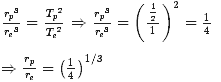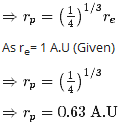Test: Gravitation - 1 - Question 15

A particle of mass 3m is located 1.00 m from a particle of mass m. Where should you put a third mass M so that the net gravitational force on M due to the two masses is exactly zero?

Detailed Solution for Test: Gravitation - 1 - Question 15

Let x be the distance of third particle from the mass 3m. then (1 - x) is the distance will be the distance from mass m. If we require that net force on another mass M be zero, then we must have the following :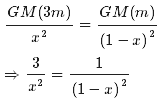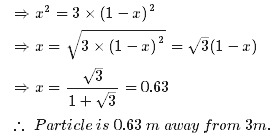Test: Gravitation - 1 - Question 16

Moon has a mass of 7.36 x 1022 kg, and a radius of 1.74 x 106 m. Calculate the acceleration due to gravity on the moon.

Detailed Solution for Test: Gravitation - 1 - Question 16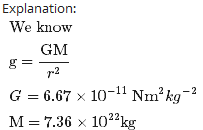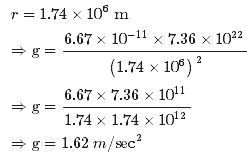Test: Gravitation - 1 - Question 17

If a film of width l is stretched in the longitudinal direction a distance d by force F, surface tension is given by

Detailed Solution for Test: Gravitation - 1 - Question 17
• Let L be the width of the film. Since the film has two surfaces, the total length along which the surface force acts on the slider is 2L.
• The surface tension S in the film is defined as the ratio of the surface force F to the length d (perpendicular to the force) along which the force acts S=F/d
• Hence, in the case, d = 2L S=F/2L
Test: Gravitation - 1 - Question 18

Titania, the largest moon of the planet Uranus, has 1/8 the radius of the earth and 1/1700 the mass of the earth. What is the acceleration due to gravity at the surface of Titania

Data: G = 6.67x10−11 N m2/kg, RE = 6.38 x 106 m, mE = 5.97 x 1024 kg

Detailed Solution for Test: Gravitation - 1 - Question 18

We know that gravitational acceleration, g = GM/R2
We know that M = Mass of earth /1700 and R = Radius of earth /8
Hence we get g = 64/1700 times the gravitational acceleration of earth
I.e. g = 64/1700 x 9.8
= 0.37 m/s2

Test: Gravitation - 1 - Question 19

Which of the following statements correct?

Detailed Solution for Test: Gravitation - 1 - Question 19

The acceleration due to gravity due to a body at point P on the surface of earth is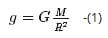Let the body is placed to point Q at a height h abovr the surface of earth, then acceleration due to gravity: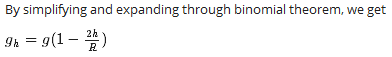From above expression we can clearly say that with increase in altitiude h , the value of acceleration due to gravity decreases.

Test: Gravitation - 1 - Question 20

How will you ‘weigh the sun’, that is estimate its mass? The mean orbital radius of the earth around the sun is 1.5 x 108 km.

Detailed Solution for Test: Gravitation - 1 - Question 20

R = Radius of Orbit of earth = 1.5 x 108 km = 1.5 x 1011m

T = time Period of earth around the sun = 365 Days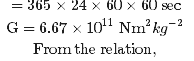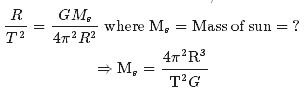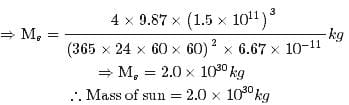## Physics Class 11

126 videos|449 docs|210 tests
 Use Code STAYHOME200 and get INR 200 additional OFF Use Coupon Code
Information about Test: Gravitation - 1 Page
In this test you can find the Exam questions for Test: Gravitation - 1 solved & explained in the simplest way possible. Besides giving Questions and answers for Test: Gravitation - 1, EduRev gives you an ample number of Online tests for practice

## Physics Class 11

126 videos|449 docs|210 tests

### How to Prepare for NEET

Read our guide to prepare for NEET which is created by Toppers & the best Teachers